# TSA MUSIC VI

LabVIEW 2014 Advanced Signal Processing Toolkit Help

Edition Date: June 2014

Part Number: 372656C-01

»View Product InfoDownload Help (Windows Only)

Owning Palette: Correlation and Spectral Analysis VIs

Computes the single-sided power spectral density (PSD) of a univariate or multivariate (vector) time series using the multiple signal classification (MUSIC) method. The MUSIC method performs a comprehensive and accurate spectral analysis on multivariate time series. Wire data to the Xt input to determine the polymorphic instance to use or manually select the instance.

Use the pull-down menu to select an instance of this VI.

 Select an instance TSA MUSIC (Waveform)TSA MUSIC (Array)TSA Vector MUSIC (Waveform)TSA Vector MUSIC (Array)

## TSA MUSIC (Waveform)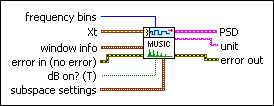frequency bins specifies the number of frequency bins for which this VI computes the single-sided power spectral density PSD. The length of the single-sided PSD is (frequency bins/2+1). The default is 1024.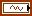Xt specifies the univariate time series.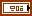window info specifies the information of the sliding window that divides the time series into subsequences.window specifies the time-domain window applied to the time series. Options include None (default), Hanning, Hamming, Blackman-Harris, Exact Blackman, Blackman, Flat Top, 4 Term B-Harris, 7 Term B-Harris, and Low Sidelobe.length specifies the length of the window. A large window generates a power spectral density (PSD) with small bias but results in a coarse PSD plot. A small window generates a smooth PSD plot but leads to large bias. The default is –1, which indicates that the window length equals the length of the input time series.overlap specifies the overlap, in percentage, of the moving window that this VI applies to the time series. This parameter determines how much data this VI reuses for the signal space matrix. A large overlap reduces the variance of the resulting power spectrum but increases computation time. The default is 50, which specifies that the overlap is half of the window length.error in describes error conditions that occur before this node runs. This input provides standard error in functionality.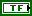dB on? specifies whether this VI returns the PSD in decibels or in a linear scale. If dB on? is TRUE, this VI returns the PSD in decibels. If dB on? is FALSE, this VI returns the PSD in a linear scale. The default is TRUE.subspace settings specifies options for the MUSIC method.noise subspace specifies the percentage of the noise subspace in the whole space, which is the combination of the signal subspace and the noise subspace. The default is –1, which means this VI calculates an appropriate value for the noise subspace.weighting specifies the weighting method for spectrum estimation.

 0 None (default) 1 Eigenvector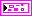PSD returns information about the single-sided power spectral density (PSD).f0 returns the lower boundary, in hertz, of the frequency range.df returns the frequency increment, in hertz.S(f) returns the magnitude of the PSD at each frequency. The value of dB on? determines the unit of measurement for this parameter.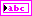unit returns the engineering unit of the PSD. You can specify an engineering unit for a time series by using the TSA Scale to EU VI.error out contains error information. This output provides standard error out functionality.

## TSA MUSIC (Array)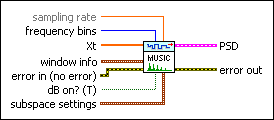sampling rate specifies the sampling rate, in hertz, of the univariate time series Xt. The default is 1.frequency bins specifies the number of frequency bins for which this VI computes the single-sided power spectral density PSD. The length of the single-sided PSD is (frequency bins/2+1). The default is 1024.Xt specifies the univariate time series.window info specifies the information of the sliding window that divides the time series into subsequences.window specifies the time-domain window applied to the time series. Options include None (default), Hanning, Hamming, Blackman-Harris, Exact Blackman, Blackman, Flat Top, 4 Term B-Harris, 7 Term B-Harris, and Low Sidelobe.length specifies the length of the window. A large window generates a power spectral density (PSD) with small bias but results in a coarse PSD plot. A small window generates a smooth PSD plot but leads to large bias. The default is –1, which indicates that the window length equals the length of the input time series.overlap specifies the overlap, in percentage, of the moving window that this VI applies to the time series. This parameter determines how much data this VI reuses for the signal space matrix. A large overlap reduces the variance of the resulting power spectrum but increases computation time. The default is 50, which specifies that the overlap is half of the window length.error in describes error conditions that occur before this node runs. This input provides standard error in functionality.dB on? specifies whether this VI returns the PSD in decibels or in a linear scale. If dB on? is TRUE, this VI returns the PSD in decibels. If dB on? is FALSE, this VI returns the PSD in a linear scale. The default is TRUE.subspace settings specifies options for the MUSIC method.noise subspace specifies the percentage of the noise subspace in the whole space, which is the combination of the signal subspace and the noise subspace. The default is –1, which means this VI calculates an appropriate value for the noise subspace.weighting specifies the weighting method for spectrum estimation.

 0 None (default) 1 EigenvectorPSD returns information about the single-sided power spectral density (PSD).f0 returns the lower boundary, in hertz, of the frequency range.df returns the frequency increment, in hertz.S(f) returns the magnitude of the PSD at each frequency. The value of dB on? determines the unit of measurement for this parameter.error out contains error information. This output provides standard error out functionality.

## TSA Vector MUSIC (Waveform)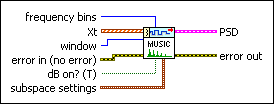frequency bins specifies the number of frequency bins for which this VI computes the single-sided power spectral density PSD. The length of the single-sided PSD is (frequency bins/2+1). The default is 1024.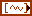Xt specifies the multivariate (vector) time series.window specifies the time-domain window applied on the time series in frequency computation.error in describes error conditions that occur before this node runs. This input provides standard error in functionality.dB on? specifies whether this VI returns the PSD in decibels or in a linear scale. If dB on? is TRUE, this VI returns the PSD in decibels. If dB on? is FALSE, this VI returns the PSD in a linear scale. The default is TRUE.subspace settings specifies options for the MUSIC method.noise subspace specifies the percentage of the noise subspace in the whole space, which is the combination of the signal subspace and the noise subspace. The default is –1, which means this VI calculates an appropriate value for the noise subspace.weighting specifies the weighting method for spectrum estimation.

 0 None (default) 1 EigenvectorPSD returns information about the single-sided power spectral density (PSD).f0 returns the lower boundary, in hertz, of the frequency range.df returns the frequency increment, in hertz.S(f) returns the magnitude of the PSD at each frequency. The value of dB on? determines the unit of measurement for this parameter.error out contains error information. This output provides standard error out functionality.

## TSA Vector MUSIC (Array)sampling rate specifies the sampling rate, in hertz, of the univariate time series Xt. The default is 1.frequency bins specifies the number of frequency bins for which this VI computes the single-sided power spectral density PSD. The length of the single-sided PSD is (frequency bins/2+1). The default is 1024.Xt specifies the multivariate (vector) time series. Each column of the 2D array represents a vector at certain time.window specifies the time-domain window applied on the time series in frequency computation.error in describes error conditions that occur before this node runs. This input provides standard error in functionality.dB on? specifies whether this VI returns the PSD in decibels or in a linear scale. If dB on? is TRUE, this VI returns the PSD in decibels. If dB on? is FALSE, this VI returns the PSD in a linear scale. The default is TRUE.subspace settings specifies options for the MUSIC method.noise subspace specifies the percentage of the noise subspace in the whole space, which is the combination of the signal subspace and the noise subspace. The default is –1, which means this VI calculates an appropriate value for the noise subspace.weighting specifies the weighting method for spectrum estimation.

 0 None (default) 1 EigenvectorPSD returns information about the single-sided power spectral density (PSD).f0 returns the lower boundary, in hertz, of the frequency range.df returns the frequency increment, in hertz.S(f) returns the magnitude of the PSD at each frequency. The value of dB on? determines the unit of measurement for this parameter.error out contains error information. This output provides standard error out functionality.